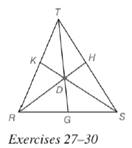Chapter 5.6, Problem 29EElementary Geometry For College St...

7th Edition
Alexander + 2 others
ISBN: 9781337614085

Solutions

Chapter
SectionElementary Geometry For College St...

7th Edition
Alexander + 2 others
ISBN: 9781337614085
Textbook Problem

Given point D in the interior of Δ R S T , suppose that R G = 3 , G S = 4 , S H = 4 , H T = 5 , and T K = 3. Find K R .To determine

To find:

The value of KR.

Explanation

Given:

Consider a point D in the interior of ΔRST and RG=3,GS=4,SH=4,HT=5,TK=3.

Theorem used:

Ceva’s theorem:

Let point D be any point in the interior of ΔABC, where E, F, and G lie on ΔABC, let BE¯,AF¯ and CG¯ be the line segments determined by D and vertices of ΔABC. Then the product of the ratios of the lengths of the segments of each of the three sides (taken in order from a given vertex of the triangle) equals 1; that is AGGBBFFCCEEA=1.

Calculation:

Let D be a point in the interior of ΔRST

Still sussing out bartleby?

Check out a sample textbook solution.

See a sample solution

The Solution to Your Study Problems

Bartleby provides explanations to thousands of textbook problems written by our experts, many with advanced degrees!

Get Started

Change 64 ft/s to mi/h.

Elementary Technical Mathematics

In Exercises 2528. solve the equation by factoring. 28. 2x4 + x2 = 1

Applied Calculus for the Managerial, Life, and Social Sciences: A Brief Approach

Find the most general antiderivative of the function. f(t) = 2 sin t 3et

Single Variable Calculus: Early Transcendentals, Volume I

83-94 Evaluate the integral. 55edx

Calculus (MindTap Course List)

For , f(x) = 0 2 3 f(3) does not exist

Study Guide for Stewart's Single Variable Calculus: Early Transcendentals, 8th

In Exercise 1320, plot the point on a set of coordinate axes. (2,5)

Finite Mathematics for the Managerial, Life, and Social Sciences